# GROUP BY Clause in MySQL

## GROUP BY Clause in MySQL with Examples

In this article, I am going to discuss the Group by Clause in the MySQL with Examples. Please read our previous article where we discussed the WHERE Clause in MySQL.

##### Group by Clause in MySQL

As the name suggests the GROUP BY clause in MySQL groups the data together. It aggregates many rows into one. The FROM and WHERE clause creates an intermediate tabular result set and the GROUP BY clause systematically groups the data. The GROUP BY clause can group the result set by one or more columns. That means the Group by Clause divides the similar type of records or data as a group and then returns. If we use group by clause in the query then we should use grouping/aggregate function such as count(), sum(), max(), min(), avg() functions.

When we implement group by clause, first the data of the table will be divided into separate groups as per the column and later aggregate function will execute on each group data to get the result. That means the first Group By clause is used to divide similar types of data as a group and then an aggregate function is applied to each group to get the required results.

##### Syntax of Group by Clause in MySQL

Following is the basic syntax to use Group by Clause in MySQL Database.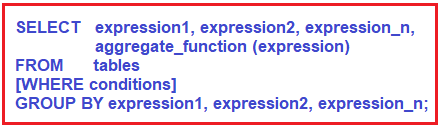##### Parameters or Arguments used in Group by Clause in MySQL:
1. expression1, expression2, expression_n: The expressions that are not encapsulated within an aggregate function must be included in the GROUP BY clause.
2. aggregate_function: The aggregate function can be SUM, COUNT, MIN, MAX, or AVG functions that we should use while we are using the Group by Clause in MySQL.
3. Tables: Tables are nothing but the name of the table or tables from which we want to retrieve the data.
4. WHERE conditions: It is optional. If you want to retrieve the data based on some conditions then you need to specify such conditions using the Where Clause.

Note: The GROUP BY clause in MySQL should be placed after the FROM and WHERE clauses. Again there must be at least one column or one expression after the GROUP BY keyword, that you want to use as criteria to group the records or rows.

##### Examples to understand Group by Clause in MySQL

We are going to use the following employee table to understand the Group By Clause in MySQL with examples.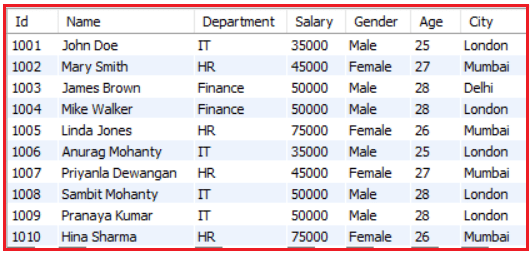Please use the below SQL Script to create the database company and employee table with the required data.

```CREATE DATABASE company;
USE company;

CREATE TABLE employee (
Id INT PRIMARY KEY,
Name VARCHAR(45) NOT NULL,
Department VARCHAR(45) NOT NULL,
Salary FLOAT NOT NULL,
Gender VARCHAR(45) NOT NULL,
Age INT NOT NULL,
City VARCHAR(45) NOT NULL
);

INSERT INTO employee (Id, `Name`, Department, Salary, Gender, Age, City) VALUES (1001, 'John Doe', 'IT', 35000, 'Male', 25, 'London');
INSERT INTO employee (Id, `Name`, Department, Salary, Gender, Age, City) VALUES (1002, 'Mary Smith', 'HR', 45000, 'Female', 27, 'Mumbai');
INSERT INTO employee (Id, `Name`, Department, Salary, Gender, Age, City) VALUES (1003, 'James Brown', 'Finance', 50000, 'Male', 28, 'Delhi');
INSERT INTO employee (Id, `Name`, Department, Salary, Gender, Age, City) VALUES (1004, 'Mike Walker', 'Finance', 50000, 'Male', 28, 'London');
INSERT INTO employee (Id, `Name`, Department, Salary, Gender, Age, City) VALUES (1005, 'Linda Jones', 'HR', 75000, 'Female', 26, 'Mumbai');
INSERT INTO employee (Id, `Name`, Department, Salary, Gender, Age, City) VALUES (1006, 'Anurag Mohanty', 'IT', 35000, 'Male', 25, 'London');
INSERT INTO employee (Id, `Name`, Department, Salary, Gender, Age, City) VALUES (1007, 'Priyanla Dewangan', 'HR', 45000, 'Female', 27, 'Mumbai');
INSERT INTO employee (Id, `Name`, Department, Salary, Gender, Age, City) VALUES (1008, 'Sambit Mohanty', 'IT', 50000, 'Male', 28, 'London');
INSERT INTO employee (Id, `Name`, Department, Salary, Gender, Age, City) VALUES (1009, 'Pranaya Kumar', 'IT', 50000, 'Male', 28, 'London');
INSERT INTO employee (Id, `Name`, Department, Salary, Gender, Age, City) VALUES (1010, 'Hina Sharma', 'HR', 75000, 'Female', 26, 'Mumbai');
```

Once you execute the above SQL statements, then it will create the company database and inside it will create the employee table as shown in the below image.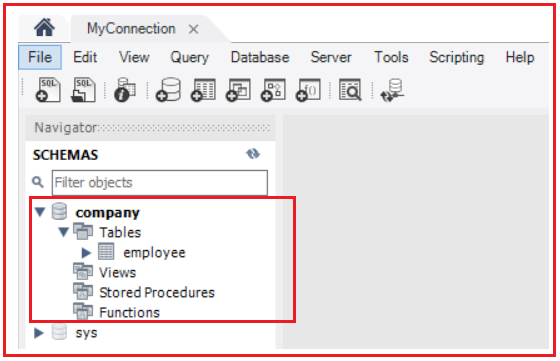##### MySQL GROUP BY Clause with the COUNT function

Now let us see some examples of using Group by Clause with COUNT aggregate function. The COUNT aggregate function in MySQL is going to return the count of the total number of records.

###### Example: Count the total number of employees in the employee table

SELECT COUNT(*) AS TotalEmployee FROM Employee;
Output: 10

As the employee table contains a total of 10 records, so the count function returns 10. As you can see, here we are not using any group by function.

###### Example: Count the total number of employees in each department of the employee table

So, here first we need to group the employees by department, and then we need to execute the count function on each group. The following SQL Query exactly does the same.

```SELECT Department, COUNT(*) AS TotalEmployee
FROM Employee
GROUP BY Department;```

When you execute the above query, you will get the below output which is showing the department name along with the number of employees of that department.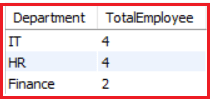##### MySQL GROUP BY Clause with the SUM function

Now let us see some examples of using Group by Clause with SUM aggregate function. The SUM aggregate function in MySQL is going to sum the value on the column on which it is operated.

###### Example: Find the total salary given to all employees

SELECT SUM(Salary) as TotalSalary FROM Employee;
Output: 510000

###### Example: Count the total salary given to each department in the employee table

Here first we need to group the employees by department and then we need to execute the SUM function on each group. And to SUM function we need to pass the Salary column. The following SQL Query exactly does the same.

```SELECT Department, SUM(Salary) as TotalSalary
FROM Employee
GROUP BY Department;```

When we execute the above query, you will get the below output which is showing the department name along with the total salary given to that department.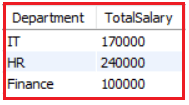##### MySQL GROUP BY Clause with MAX and MIN function

Now let us see some examples of using Group by Clause with MAX and MIN aggregate function. The MAX aggregate function in MySQL is going to return the max value whereas the MIN aggregate function is going to return the Min value.

###### Example: Find the Highest salary in Each Department of the Organization

Here first we need to group the employees by department and then we need to execute the MAX function on each group. And to the MAX function, we need to pass the Salary column as shown in the below SQL Query.

```SELECT Department, MAX(SALARY) as MaxSalary
FROM Employee
GROUP BY Department;```

When you execute the above SQL query, it will give you the below output which is showing the department name along with the highest salary of that department.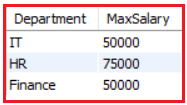###### Example: Find the Lowest salary in Each Department

Here first we need to group the employees by department and then we need to execute the MIN Aggregate function on each group. And to the MIN function, we need to pass the Salary column as shown in the below SQL Query.

```SELECT Department, MIN(SALARY) as MinSalary
FROM Employee
GROUP BY Department;```

When you execute the above SQL statement, it will give you the following output which is showing the department name along with the lowest salary of that department.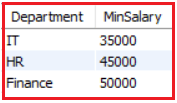##### GROUP By Clause with Multiple Columns in MySQL:

It is also possible to use Group by Columns on Multiple Columns in MySQL. In that case, first, data in the table is divided based on the first column of the group by clause and then each group is subdivided based on the second column of the group by clause and then the group function is applied to each inner group to get the result.

###### Example: Find the number of employees working in each Gender per department

So, what our requirement is, first we need to group the employee by the department and then we need to group the employee by salary present in each department.

```SELECT Department, Salary, COUNT(*) AS EmployeeCount
FROM Employee
GROUP BY Department, Salary
ORDER BY Department;```

When we execute the above query, it gives us the below output.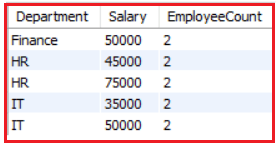###### Example: Find the total salaries and the total number of employees by City, and by gender

Here, we need to group by City first and then by Gender. Then we need to apply the SUM Aggregate function on the Salary column to calculate the total sum and the Count Aggregate function on the Id column to find the total number of employees.

```SELECT City, Gender,
SUM(Salary) as TotalSalary,
COUNT(ID) as TotalEmployees
FROM Employee
GROUP BY CITY, Gender;```

When we execute the above query, it gives us the below output.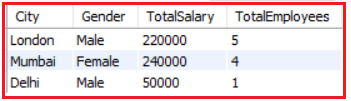##### MySQL GROUP BY Clause with AVG function

Now let us see one example of using Group by Clause with AVG aggregate function. The ACG aggregate function in MySQL is going to return the average value of the column on which it is operated.

###### Example: Find the average salary of each department

Here first we need to group the employees by department and then we need to execute the AVG Aggregate function on each group. And to AVG Aggregate function we need to pass the Salary column as shown in the below SQL Query.

```SELECT Department, AVG(Salary) as AverageSalary
FROM Employee
GROUP BY Department;```

When we execute the above query, you will get the below output which is showing the department name along with the average salary of that department.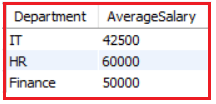Note: When the aggregate function is applied to a group it returns only a single value but each group can return a value. Use Group By clause only on a column that contains duplicate values, never applies it on unique columns.

1.